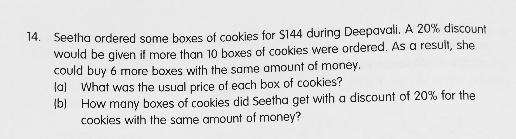# QuestionAnyone help me with attached question

Thanks

20% of \$144 = 144 × 0.2 = \$28.80

She could buy 6 more with \$28.80.

Each box  -> 28.80 ÷ 6 = \$4.80 (discounted price)

(a) Usual price -> 4.80 ÷ 0.8 = \$6.00

(b) Number of boxes bought at discounted price -> \$144 ÷ 4.80 = 30

Thank you so much

0 Replies 0 Likes

Let the  number of boxes and price of each box be 1u and 1p respectively.
usual price of each box if 1u <= 10 ——- 1up

1u x 1p = 1up ——- 144
0.8(1u + 6) x 1p ——- 144
1up + 6p = 144 + 6p ——- 144/0.8 = 180
1p ——- (180 – 144)/6 = 6

(b)
144/6 = 24
24 + 6 = 30

Ans : (a) \$6; (b) 30 boxes.

0 Replies 0 Likes